# Bihar Board 12th Maths Important Questions Short Answer Type Part 2

BSEB Bihar Board 12th Maths Important Questions Short Answer Type Part 2 are the best resource for students which helps in revision.

## Bihar Board 12th Maths Important Questions Short Answer Type Part 2

Question 1.
∫cos4x dx = ∫$$\frac {(2 cos² x)²}{4}$$ dx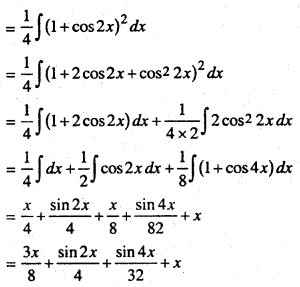Question 2.
∫$$\frac { cosx dx)}{(1 – sin x)(2 – sin x)}$$Question 3.
∫$$\frac {sec²(log x)}{x}$$ dx
Let I = ∫$$\frac {sec²(log x)}{x}$$ dx
Let log x = z, Diff. with repect for, we get
$$\frac {1}{x}$$ = $$\frac {dz}{dx}$$ = $$\frac {dx}{x}$$ = dz
Putting this value in (1) we get
I = ∫sec² z dz = tan z + c = tan(log x )x.

Question 4.
Integrate the following
(i) ∫$$\frac {sin √x}{√x}$$ dx
(ii) ∫$$\frac {cos x}{\sqrt{1-sin x}}$$ dx
(iii) ∫$$\frac {dx}{1+e^{-x}}$$ dx
Let √x = t ⇒ $$\frac {1}{2√x}$$ = dx = dt ⇒ $$\frac {dx}{√x}$$= 2 dt
∴ I = 2∫sin t dt – 2 cos t + k
= k – 2 cos√x.

(ii) Let 1 + sin x = m ⇒ cosx ax = am.
∴ ∫$$\frac {cos x}{\sqrt{1-sin x}}$$ dx = ∫$$\frac {dm}{√m}$$ = 2$$\sqrt {m + C}$$ = 2$$\sqrt {1+5mx}$$ + CLet + ex = z ⇒ ex = dx = dz.
∴ ∫$$\frac {dz}{z}$$ = log z + c = log(1 + ex) + C

Differential Equation

Question 1.
determine order and degree of following differential equations:
(i) $$\frac {d^4y}{dx^4}$$ + sin (y”’) = 0
(ii) ($$\frac {ds}{dt}$$)4 + 3s $$\frac {d²s}{dt²}$$ = 0
(i) The highest order derivative present in the given differential equation is $$\frac {d^4y}{dx^4}$$. So its order is 4.
but degree is not define.

(ii) The highest order derivative present in the given differential equation
is $$\frac {d²s}{dt²}$$, so its order is 2 and the highest power of $$\frac {d²s}{dt²}$$ is one.
degree = 1

Question 2.
Form a differential equation of family of curve. y² = a(a² – x²)
Given equation
y² = a(a² – x²)
Diff. w.r. to x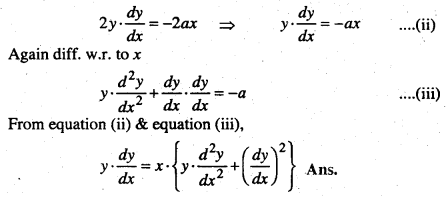Question 3.
Form a differential equation of the family of curve.
y² + y² = 2ax
Given equation
y² + y² = 2ax ……….. (i)
Diff. w.r. to x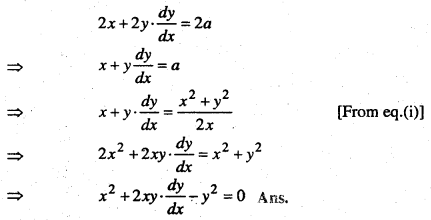Vector Algebra

Question 1.
For any two vectors $$\vec {a}$$ and $$\vec {b}$$?
$$\vec {a}$$ + $$\vec {b}$$ = $$\vec {b}$$ + $$\vec {a}$$
Consider the parallelogram ABCD.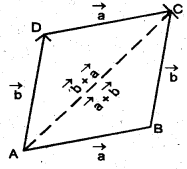Let $$\vec {AB}$$ = $$\vec {a}$$ and $$\vec {BC}$$ = $$\vec {b}$$ then using the triangle law, from triangle ABC,
We have,
$$\vec {AC}$$ = $$\vec {a}$$ + $$\vec {b}$$
Now, since the opposite sides of a parallelogram are equal and parallel, we have,
$$\vec {AD}$$ = $$\vec {BC}$$+ $$\vec {b}$$ and
$$\vec {DC}$$ = $$\vec {AB}$$ = $$\vec {a}$$
Again using triangle law, from triangle ADC, We have
$$\vec {AC}$$ = $$\vec {AD}$$ + $$\vec {DC}$$ = $$\vec {b}$$ + $$\vec {a}$$
Hence, $$\vec {a}$$ + $$\vec {b}$$ = $$\vec {b}$$ + $$\vec {a}$$

Question 2.
Find unit vector in the direction of vector :
$$\vec {a}$$ = 2$$\hat {i}$$ + 3$$\hat {j}$$ + $$\hat {k}$$
The unit vector in the direction of a vector $$\vec {a}$$ is given by: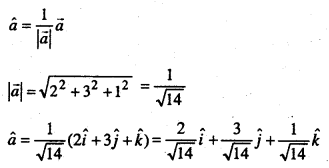Question 3.
Find a vector in the direction of vector $$\vec {a}$$ = $$\hat {i}$$ – 2$$\vec {j}$$ that has magnitude.
The unit vector in the direction of the given vector $$\vec {a}$$ is.
$$\hat {a}$$ = $$\frac {1}{|\vec {a}|}$$ $$\vec {a}$$ = $$\frac {1}{√5}$$ ($$\hat {i}$$ + 2$$\hat {j}$$) $$\frac {1}{√5}$$ $$\hat {i}$$ – $$\frac {2}{√5}$$ $$\hat {j}$$
∴ The vector having magnitude equal to 7 and in the direction of $$\vec {a}$$ is
7$$\hat {a}$$ = 7($$\frac {1}{√5}$$$$\hat {i}$$ – $$\frac {2}{√5}$$$$\hat {j}$$) = $$\frac {7}{√5}$$ $$\hat {i}$$ – $$\frac {14}{√5}$$$$\hat {j}$$

Question 4.
Show that the point A(2$$\hat {i}$$ – $$\hat {j}$$ + $$\hat {k}$$), B($$\hat {i}$$ – 3$$\hat {j}$$ – 5$$\hat {k}$$), C(3$$\hat {i}$$ – 4$$\hat {j}$$ – 4$$\hat {k}$$) are the vertices of a right angled triangle.
We have
$$\vec {AB}$$ = (1 – 2)$$\hat {i}$$ + (-3 + 1)$$\hat {j}$$ + (-5 – 1)$$\hat {k}$$ = –$$\hat {i}$$ – 2$$\hat {j}$$ – 6$$\hat {k}$$
$$\vec {BC}$$ = (3 – 1)$$\hat {i}$$ +(-4 + 3)$$\hat {j}$$ + (-4 +5)$$\hat {k}$$ = 2$$\hat {i}$$ – $$\hat {j}$$ + $$\hat {k}$$
$$\vec {CA}$$ = (2 – 3)$$\hat {i}$$ +(-4 + 4)$$\hat {j}$$ + (1 + 4)$$\hat {k}$$ = –$$\hat {i}$$ + 3$$\hat {j}$$ + 5$$\hat {k}$$.
Further, note that
|$$\vec {AB}$$| = 41 = 6 + 35 = |$$\vec {BC}$$|² + |$$\vec {CA}$$|²
Hence, the triangle is a right angled triangle,

Question 5.
If the position vectors of the points P and Q are – $$\vec {i}$$ + 2$$\vec {j}$$ + 3$$\vec {k}$$ and 7$$\vec {i}$$ – 6$$\vec {k}$$ respectively, find the direction cosines of $$\vec {PQ}$$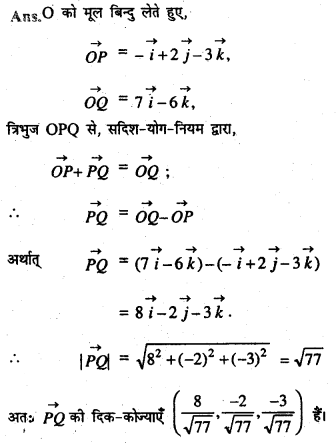Question 6.
यदि (If) \begin{aligned} \overrightarrow{r_{1}} &=3 \vec{i}-2 \vec{j}+\vec{k} \\ \overrightarrow{r_{2}} &=2 \vec{i}-4 \vec{j}-3 \vec{k} \\ \overrightarrow{r_{3}} &=-\vec{i}+2 \vec{j}+2 \vec{k} \end{aligned}
तो निम्नांकिता का मापांक ज्ञात करें (Find the modulus of the following);
$$2 \overrightarrow{r_{1}}-3 \overrightarrow{r_{2}}-5 \overrightarrow{r_{3}}$$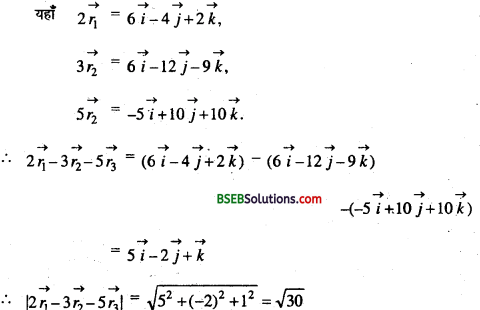Question 7.
Prove that $$2 \vec{i}- \vec{j}+ \vec{k} \vec{b}= \vec{i}-3 \vec{j}-5 \vec{k} \vec{c}=3 \vec{i}-4 \vec{j}-4 \vec{k}$$ are the sides of a right angled triangle
चूँकि $$\vec{a}+\vec{b}=(2 \vec{i}-\vec{j}+\vec{k})+(\vec{i}-3 \vec{j}-5 \vec{k})$$
= $$3 \vec{i}-4 \vec{j}-4 \vec{k}=\vec{c}$$
अतः $$\vec{a}, \vec{b}, \vec{c}$$ एक त्रिभुज बनाते है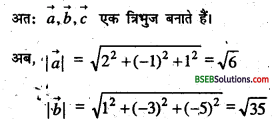Question 8.
If $$\vec{a}, \vec{b}, \vec{c}$$ are three vectors and $$a+\vec{b}+\vec{c}=\overrightarrow{0}$$ prove that $$\vec{a} \times \vec{b}=\vec{b} \times \vec{c}=\vec{c} \times \vec{a}$$
Proof:
$$\vec{a}+\vec{b}+\vec{c}=\overrightarrow{0}$$ ……………….. (1)
Taking is crossproduct with $$\vec{a}$$ on left, we’veAgain taking cross product of (1) with $$\vec{b}$$ on left, we get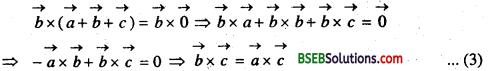From (2) and (3), weve $$\vec{a} \times \vec{b}=\vec{b} \times \vec{c}=\vec{c} \times \vec{a}$$

Question 9.
Find the projection of the vector $$\vec{a}=2 \hat{i}+3 \hat{j}+2 \hat{k}$$ on the vector
$$\vec{b}=\hat{i}+2 \hat{j}+\hat{k}$$
Solution:
The projection of vector $$\vec{a}$$ ón the vector $$\vec{b}$$ is given by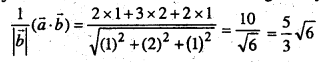Question 10.
Show that the point A($$-2 \hat{i}+3 \hat{j}+5 \hat{k}$$).B($$\hat{i}+2 \hat{j}+3 \hat{k}$$)and ($$7 \hat{i}-\hat{k}$$) are collancar. .
Solution:
We have
$$\vec {AB}$$ = (1 + 2)$$\hat {i}$$ + (2 – 3)$$\hat {j}$$ + (3 – 5)$$\hat {k}$$ = 3$$3\hat {i}$$ – $$\hat {j}$$ – 2$$\hat {k}$$
$$\vec {BC}$$ = (7 – 1)$$\hat {i}$$ +(0 – 2)$$\hat {j}$$ + (-1 -3)$$\hat {k}$$ = 6$$\hat {i}$$ – 2$$\hat {j}$$ – 4$$\hat {k}$$
$$\vec {CA}$$ = (7 + 2)$$\hat {i}$$ +(0 – 3)$$\hat {j}$$ + (-5 – 5)$$\hat {k}$$ = 9$$\hat {i}$$ 3$$\hat {j}$$ – 6$$\hat {k}$$.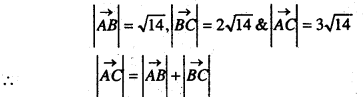Hence the points A, B and Care collinear.Question 11.
Find the area of a parallelogram whose adjacent sides are given by the vectors $$\vec{a}=3 \hat{i}+\hat{j}+4 \hat{k}$$ and $$\vec{b}=\hat{i}-\hat{j}+\hat{k}$$
Solution:
The area of a parallelogram with $$\vec{a}$$ and $$\vec{b}$$ as its adjacent sides is given by $$|\vec{a} \times \vec{b}|$$
$$\vec{a} \times \vec{b}=\left|\begin{array}{ccc} \hat{i} & \hat{j} & \hat{k} \\ 3 & 1 & 4 \\ 1 & -1 & 1 \end{array}\right|=5 \hat{i}+\hat{j}-4 \hat{k}$$
$$\vec{a} \times \vec{b}=\sqrt{25+1+6}=\sqrt{42}$$
Hence the required area $$\sqrt{42}$$

Probability

Question 1.
If P(A) = $$\frac { 7 }{ 13 }$$ $$\frac { 9 }{ 13 }$$ and P(B) = $$\frac { 4 }{ 13 }$$ and P(A∩B) = evaluate P(A/B)
Solution:
We have P(A/B) = $$\frac{P(A \cap B)}{P(B)}=\frac{\frac{4}{13}}{\frac{9}{13}}=\frac{4}{9}$$

Question 2.
A family has two children. What is the probabiity that both the children are boys given that at lest one of them is a boy?
Solution:
Let b stand for boy and g for girl.
The sample space of the experiment is
S = ((b, b),(g, b),(b, g), (g, g))
Let E and F denote the following events.
E: ‘both the children are boys’
F:’ atleast on the child is a boy’ ,
Then, E = {(b,b)} and F = {(b,b),(g,b),(b,gi}
Noi, E ∩ F = {(b, b)} .
Thus P(F) = $$\frac { 3 }{ 4 }$$ and P(E∩F) = $$\frac { 1 }{ 4 }$$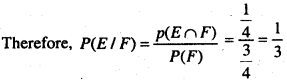Question 3.
A die is thrown If E is the eient the number appearing is a multiple of 3’ and F be the event ‘the number appearing is evem Then find whether E and F are independent.
Solution:
We know the sample space is S = { 1, 2, 3,4, 5, 6}
Now E = {3,6},
F = {2,4,6) and E ∩ F = {6}
Then, P(E) = $$\frac{2}{6}=\frac{1}{3}$$
P(F) = $$\frac{3}{6}=\frac{1}{2}$$
and P(E∩F) = $$\frac{1}{6}$$
Clearly P(E∩F)= P(E).P(F)
Hence E and F are independent events.Question 4.
An unbaised dic is thrown twice, Let the event A be odd number on the first throw and B thc event ‘odd number on the second throw.
Check the independence of the events A and B.
Solution:
If all the 36 elementary events of the experiment are consided to be equally likely.
We have,
P(A) = $$\frac{18}{36}=\frac{1}{2}$$
and = $$\frac{18}{36}=\frac{1}{2}$$
Also P(A ∩B) = P (odd number on both throws)
$$\frac{9}{36}=\frac{1}{4}$$
Now, P(A)P(B) = $$\frac{1}{2} \times \frac{1}{2}=\frac{1}{4}$$
CIçarly, P(A∩B) = P(A) x P(B)
Thus A and B are independènt events.